# Past Year Questions: Fluid Kinematics Notes | Study Topic wise GATE Past Year Papers for Civil Engineering - Civil Engineering (CE)

## Civil Engineering (CE): Past Year Questions: Fluid Kinematics Notes | Study Topic wise GATE Past Year Papers for Civil Engineering - Civil Engineering (CE)

The document Past Year Questions: Fluid Kinematics Notes | Study Topic wise GATE Past Year Papers for Civil Engineering - Civil Engineering (CE) is a part of the Civil Engineering (CE) Course Topic wise GATE Past Year Papers for Civil Engineering.
All you need of Civil Engineering (CE) at this link: Civil Engineering (CE)

Question 1.  The velocity field in a flow system is given by v = 2i+ (x + y)j + (xyz)k. The acceleration of the fluid at (1, 1,2) is    [2019 : 1 Mark, Set-II]
(a) 4i + 12k
(b) 2i + 10k
(c) j + k
(d) 4j + 10k
(d)
Solution: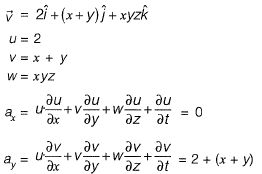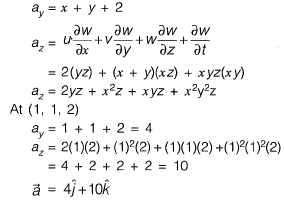Question 2. A flow field is given by u = y2, v = - xy, w = 0.Value of the z-component of the angular velocity (in radians per unit time, up to two decimal places) at the point (0, -1 ,1 ) is _____ .     [2018 : 1 Mark, Set-I]
Solution: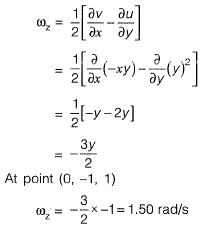Question 3.  The velocity components of a two dimensional plane motion of a fluid are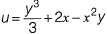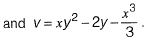The correct statement is:    [2015 : 2 Marks, Set-II]
(a) Fluid is incompressible and flow is irrotational
(b) Fluid is incompressible and flow is rotational
(c) Fluid is compressible and flow is irrotational
(d) Fluid is compressible and flow is rotational
(a)
Solution:
Apply continuity equation for 2D in compressible flow,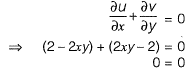Hence, incompressible flow.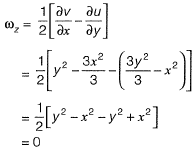Hence, flow is irrotational.

Question 4.  A nozzle is so shaped that the average flow velocity changes linearly from 1.5 m/s at the beginning to 15 m/s at its end in a distance of 0.375 m. The magnitude of the convective acceleration (in m/s2) at the end of the nozzle is _______     [2015 : 1 Mark, Set-II]
Solution:
Convective acceleration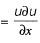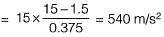Question 5. In a two-dimensional steady flow field, in a certain region of the x-y plane, the velocity component in the x-direction is given by vx = x2 and the density varies as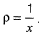Which of the following is a valid expression for the velocity component in the y-direction, vy?
(a)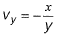(b)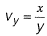(c)  vy = - xy
(d)  vy = x
(c)
Solution: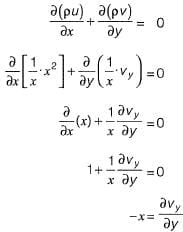On integrating both sides,
vy=-x

Question 6.  A particle moves along a curve whose parametric equations are: x = t3 + 2t, y=-3e-2t and z = 2 sin (5t), where x, y and z show variations of the distance covered by the particle (in cm) with time t(in s). The magnitude of the acceleration of the particle (in cm/s2) at t = 0 is ________ .     [2014 : 2 Marks, Set-I]
Solution: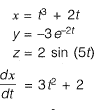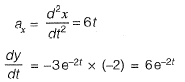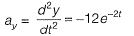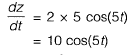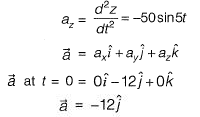⇒Magnitude of acceleration at t =0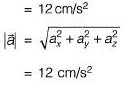Question 7. A plane flow has velocity components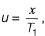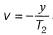and w = 0 along x, y and z directions respectively, where T1 (≠ 0) and T2 (≠ 0) are constants having the dimension of time. The given flow is incompressible if     [2014 : 1 Mark, Set-II]
(a) T1 = - T2
(b)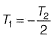(c)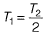(d) T1 = T2
(d)
Solution:
For a flow to exist, continuity should be satisfied.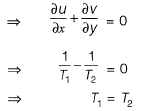Question 8. An incompressible homogeneous fluid is flowing steadily in a variable diameter pipe having the large and small diameters as 15 cm and 5 cm, respectively. If the velocity at a section at the 15 cm diameter portion of the pipe is 2.5 m/s, the velocity of the fluid (in m/s) at a section falling in 5 cm portion of the pipe is _______ .     [2014 : 1 Mark, Set-I]
Solution: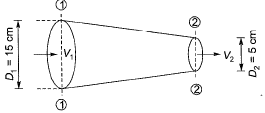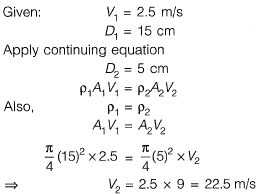Question 9.  For a 2-D flow field, the stream function ψ is given as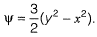The magnitude of discharge occurring between the stream line passing through points (0,3) and (3, 4) is    [2013 : 1 Mark]
(a) 6 units
(b) 3 units
(c) 1.5 units
(d) 2 units
Solution: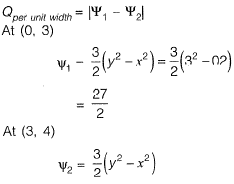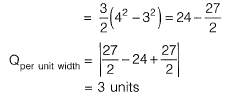The document Past Year Questions: Fluid Kinematics Notes | Study Topic wise GATE Past Year Papers for Civil Engineering - Civil Engineering (CE) is a part of the Civil Engineering (CE) Course Topic wise GATE Past Year Papers for Civil Engineering.
All you need of Civil Engineering (CE) at this link: Civil Engineering (CE)Use Code STAYHOME200 and get INR 200 additional OFF

## Topic wise GATE Past Year Papers for Civil Engineering

67 docs

Track your progress, build streaks, highlight & save important lessons and more!

,

,

,

,

,

,

,

,

,

,

,

,

,

,

,

,

,

,

,

,

,

;